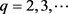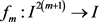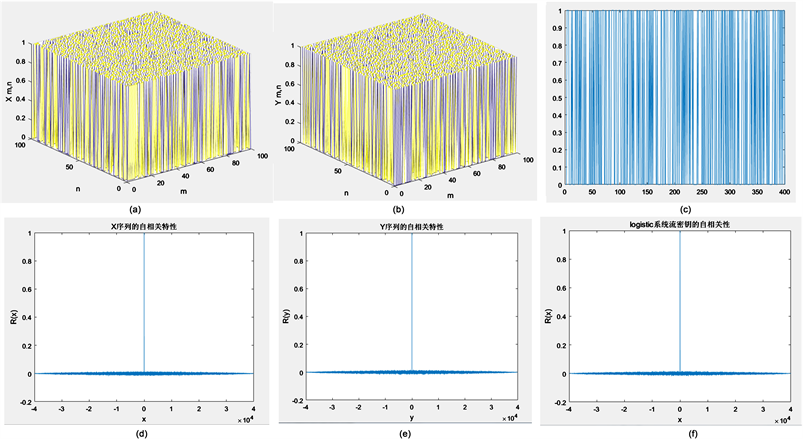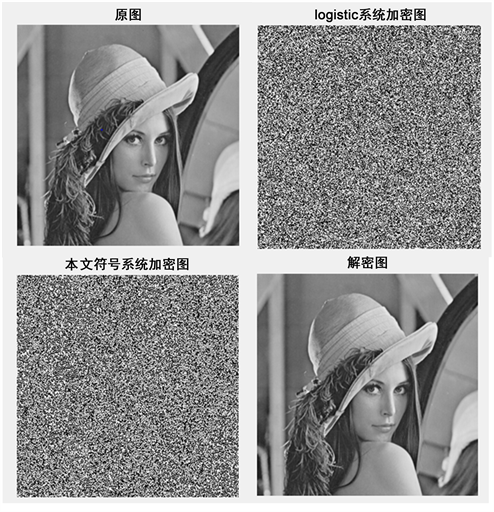﻿ 基于二维时变符号混沌系统的流密码算法设计

# 基于二维时变符号混沌系统的流密码算法设计Design of a Stream Cipher Algorithm Based on Time-Varying Symbolic Chaotic Dynamical Systems

Abstract: This paper studies chaos of a class of two-dimensional time-varying generalized symbolic systems, gives a construction example of a special time-varying generalized symbolic chaotic system, and analyses the pseudorandomicity of solutions of this system. At the same time, based on this system and m sequences, this paper designs a stream cipher algorithm and simulates its encryption effect in digital image. Simulation shows that the designed algorithm has good encryption effects.

1. 引言

$\left\{\begin{array}{l}{x}_{m+1,n}=f\left(m,{x}_{m,n},{y}_{m,n},{x}_{m,n+1}\right)\\ {y}_{m+1,n}=g\left(m,{y}_{m,n},{x}_{m,n},{y}_{m,n+1}\right)\end{array}$ (1)

$Z=\left\{\cdots ,-1,0,1,\cdots \right\}$${N}_{t}=\left\{t,t+1,\cdots \right\}$$t\in Z$ 。记 $\Omega =\left\{\left(0,n\right)|n\in {N}_{0}\right\}$ ，则对任意定义在 $\Omega$ 上的序列 $\varphi =\left\{{\varphi }_{0,n}\right\}$$\phi =\left\{{\phi }_{0,n}\right\}$ ，一定存在二维离散时空序列 $\left(x,y\right)={\left\{\left({x}_{m,n},{y}_{m,n}\right)\right\}}_{m,n=0}^{\infty }$ 满足(1)，且 ${x}_{m,n}={\varphi }_{m,n}$${y}_{m,n}={\phi }_{m,n}$ ，对任意 $\left(m,n\right)\in \Omega$ 。称 $\left(x,y\right)$ 为系统(1)初值为 $\left(\varphi ,\phi \right)$ 的一个解。参照文献  ，当 $I={Z}_{q}=\left\{0,1,\cdots ,q-1\right\}$$q\in {N}_{2}$ 时，可将系统(1)称为(二维时变)(广义)符号动力系统。现有文献对时变符号系统研究很少，系统(1)的混沌性还没有文献研究过。

$x={\left\{\left({x}_{m,n},{y}_{m,n}\right)\right\}}_{m,n=0}^{\infty }$ 是系统(1)的一个解，其中， ${x}_{0,n},{y}_{0,n}\in I$$n\in {N}_{0}$ 。记

${x}_{m}={\left\{\left({x}_{m,n},{y}_{m,n}\right)\right\}}_{n=0}^{\infty }$ , $m\in {N}_{0}$ , (2)

${I}_{2}^{\infty }=\left\{{\left\{{\left({a}_{n},{b}_{n}\right)}^{\text{T}}\right\}}_{n=0}^{\infty }=\left(\begin{array}{ccccc}{a}_{0}& {a}_{1}& \cdots & {a}_{n}& \cdots \\ {b}_{0}& {b}_{1}& \cdots & {b}_{n}& \cdots \end{array}\right)|{a}_{i},{b}_{i}\in I,i=0,1,\cdots \right\}$ . (3)

$d\left(x,y\right)=\underset{n=0}{\overset{\infty }{\sum }}\frac{|{x}_{1,n}-{y}_{1,n}|+|{x}_{2,n}-{y}_{2,n}|}{{2}^{n}}$ , (4)

${x}_{m+1}={\left\{{\left(f\left(m,{x}_{m,n},{y}_{m,n},{x}_{m,n+1}\right),g\left(m,{y}_{m,n},{x}_{m,n},{y}_{m,n+1}\right)\right)}^{\text{T}}\right\}}_{n=0}^{\infty }={g}_{m+1}\left({x}_{m}\right)$ , (5)

${G}_{n}\left(x\right)={g}_{n}\left({g}_{n-1}\left(\cdots \left({g}_{1}\left(x\right)\right)\cdots \right)\right)={g}_{n}\circ \cdots \circ {g}_{2}\circ {g}_{1}\left(x\right)$ , ${G}_{0}\left(x\right)=x$ . (6)

2. 一类二维时变广义符号混沌系统

$I={Z}_{q}=\left\{0,1,\cdots ,q-1\right\}$$f:{N}_{0}×{I}^{3}\to I$$g:{N}_{0}×{I}^{3}\to I$ 定义如下：

$f\left(m,{x}_{0},{y}_{0},{x}_{1}\right)={a}_{0,m}{x}_{0}+{a}_{1,m}{y}_{0}+a{x}_{1}\mathrm{mod}q={a}_{0,m}{x}_{0}\oplus {a}_{1,m}{y}_{0}\oplus a{x}_{1}$ , (7)

$g\left(m,{y}_{0},{x}_{0},{y}_{1}\right)={b}_{0,m}{y}_{0}\oplus {b}_{1,m}{x}_{0}\oplus b{y}_{1}$ , ${x}_{0},{x}_{1},{y}_{0},{y}_{1}\in I$$m\in {N}_{0}$ , (8)

$\left\{\begin{array}{l}{x}_{m+1,n}=f\left(m,{x}_{m,n},{y}_{m,n},{x}_{m,n+1}\right)={a}_{0,m}{x}_{m,n}\oplus {a}_{1,m}{y}_{m,n}\oplus a{x}_{m,n+1}\\ {y}_{m+1,n}=g\left(m,{y}_{m,n},{x}_{m,n},{y}_{m,n+1}\right)={b}_{0,m}{y}_{m,n}\oplus {b}_{1,m}{x}_{m,n}\oplus b{y}_{m,n+1}\end{array}$ (9)

${x}_{m+1}={g}_{m+1}\left({x}_{m}\right)$ ,  , ${x}_{m}={\left\{\left({x}_{m,n},{y}_{m,n}\right)\right\}}_{n=0}^{\infty }$ , $m\in {N}_{0}$ , (10)

${g}_{m+1}\left(\alpha \right)={\left\{\left({a}_{0,m}{u}_{n}\oplus {a}_{1,m}{v}_{n}\oplus a{u}_{n+1},{b}_{0,m}{v}_{n}\oplus {b}_{1,m}{u}_{n}\oplus b{v}_{n+1}\right)\right\}}_{n=0}^{\infty }$ . (11)

${g}_{s+mp-1}\circ {g}_{s+mp-2}\circ \cdots \circ {g}_{s}={g}_{t+mp-1}\circ {g}_{t+mp-2}\circ \cdots \circ {g}_{t}$ . (12)

$\left\{x={\left\{\left({x}_{1,n},{y}_{1,n}\right)\right\}}_{n=0}^{\infty }|{x}_{1,i}={s}_{i},{y}_{1,i}={t}_{i},i\in {Z}_{M};{x}_{1,j},{y}_{1,j}\in I,j\notin {Z}_{M}\right\}\subseteq {B}_{\theta }\left(\alpha \right)$ ,

 . (13)

${G}_{1}\left(x\right)={\left\{\left({a}_{0,0}{x}_{n}\oplus {a}_{1,0}{y}_{n}\oplus a{x}_{n+1},{b}_{0,0}{y}_{n}\oplus {b}_{1,0}{x}_{n}\oplus b{y}_{n+1}\right)\right\}}_{n=0}^{\infty }={\left\{{x}_{n}^{\left(1\right)}\right\}}_{n=0}^{\infty }={x}^{\left(1\right)}$ ,

${G}_{m}\left(x\right)={g}_{m}\left({x}^{\left(m-1\right)}\right)={g}_{m}\left(\cdots \left({g}_{1}\left(x\right)\cdots \right)\right)={\left\{{x}_{n}^{\left(m\right)}\right\}}_{n=0}^{\infty }={x}^{\left(m\right)}$ , ${x}^{\left(0\right)}=x$ ,

${x}^{\left(m\right)}={\left\{{x}_{n}^{\left(m\right)}=\left({f}_{m-1}\left({x}_{n},\cdots ,{y}_{n+m-1}\right)\oplus {a}^{m}{x}_{n+m},{h}_{m-1}\left({x}_{n},\cdots ,{y}_{n+m-1}\right)\oplus {b}^{m}{y}_{n+m}\right)\right\}}_{n=0}^{\infty }$ , (14)${h}_{m}:{I}^{2\left(m+1\right)}\to I$ 是按递推方式由G唯一决定的两列函数，对任意 $m,n\in {N}_{0}$

${f}_{m-1}\left({x}_{n},\cdots ,{y}_{n+m-1}\right)\oplus {a}^{m}r=c$ , ${h}_{m-1}\left({x}_{n},\cdots ,{y}_{n+m-1}\right)\oplus {b}^{m}h=c$ . (15)

${f}_{M-1}\left({r}_{n},\cdots ,{k}_{n+M-1}\right)\oplus {a}^{M}{r}_{n+M}={u}_{n}$ , ${h}_{M-1}\left({r}_{n},\cdots ,{k}_{n+M-1}\right)\oplus {b}^{M}{k}_{n+M}={v}_{n}$ , $n=0,1,2,\cdots$ .

${x}_{m+1,n}={a}_{0,m}{x}_{m,n}\oplus {a}_{1,m}{y}_{m,n}\oplus {x}_{m,n+1}$ , ${y}_{m+1,n}={b}_{0,m}{y}_{m,n}\oplus {b}_{1,m}{x}_{m,n}\oplus 3{y}_{m,n+1}$ , (16)Figure 1. Confusion and correlation diagram of Solutions

1) 选择常见的一副数字灰度图像作为明文，利用Matlab语言可以将该图像表示为一个数字矩阵 $I={\left({m}_{ij}\right)}_{256×256}$ ，其中，每个明文数值 ${m}_{ij}\in {Z}_{256}=\left\{0,1,\cdots ,255\right\}$

2) 先选取某个JK触发器序列作为系统(16)的初始值，再利用系统(16)的某个解 $x={\left\{{x}_{m,n}\right\}}_{m,n=0}^{\infty }$ 计算出密钥流序列，其中，需要将二元解序列 ${\left\{{x}_{m,n}\right\}}_{m,n=0}^{\infty }$ 转化为在0~255中取值的密钥流序列；

3) 加密变换： ${c}_{ij}={x}_{ij}\oplus {m}_{ij}$ ，其中， ${c}_{ij}$ 表示密文数值， $\oplus$ 表示逐比特异或运算；

4) 解密变换： ${m}_{ij}={x}_{ij}\oplus {c}_{ij}$Table 1. Three common random number test resultsFigure 2. Encryption and decryption effect diagramTable 2. Correlation between adjacent pixels between original and encrypted graphs

3. 小结

NOTES

*通讯作者。

 Devaney, R.L. (1989) An Introduction to Chaotic Dynamical Systems. 2nd Edition, Addision-Wesley, New York.

 Chen, G., Tian, C.J. and Shi, Y.M. (2005) Stability and Chaos in 2-D Discrete Systems. Chaos, Solitons and Fractals, 25, 637-647.
https://doi.org/10.1016/j.chaos.2004.11.058

 Tian, C.J. (2017) Chaos in the Sense of Devaney for Two-Dimensional Time-Varying Generalized Symbolic Dynamical Systems. Inter. J. Bifurcation and Chaos, 27, 1750060.
https://doi.org/10.1142/S0218127417500602

 Tian, C.J. and Chen, G. (2006) Chaos of a Sequence of Maps in a Metric Space. Chaos, Solitons and Fractals, 28, 1067-1075.
https://doi.org/10.1016/j.chaos.2005.08.127

 田传俊, 陈关荣. 广义符号动力系统的混沌性[J]. 应用数学学报, 2008, 31(3): 440-446.

 田传俊, 李佳佳, 曾泉, 刘明刚. 时变广义符号动力系统的混沌性及其在流密码中的应用[J]. 网络空间安全, 2016, 7(9-10): 33-36.

 田传俊, 刘明刚, 郝红建, 李佳佳. 二维时变离散时空系统的混沌性及其在流密码中的应用[J]. 信息安全与技术, 2015(7): 71-75.

 Ye, G.D., Pan, G., Huang, X.L., et al. (2018) A Chaotic Image Encryptiong Algorithm Based on Information Entropy. International Journal of Bifurcation and Chaos, 28, 1850010.
https://doi.org/10.1142/S0218127418500104

 Hua, Z.Y. and Zhou, Y.C. (2016) Image Encryption Using 2D Lo-gistic-Adjusted-Sine Map. Information Sciences, 339, 237-253.
https://doi.org/10.1016/j.ins.2016.01.017

 李大为, 冯登国, 陈华, 等, 编. 随机性检测规范[S]. 国家密码管理局, 2009.

Top